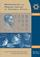Mathematical and Physical Journal
for High Schools
Issued by the MATFUND Foundation
 Already signed up? New to KöMaL?# English Issue, December 2002

Previous pageContentsNext pageORDER FORM

## Mathematics and physics quiz

(from the Conference of KöMaL)

The questions are based on the suggestions of I. Varga and J. Pataki.)

1. The foci of an ellipse are F1(9,20) and F2(49,55). The ellipse touches the x-axis. How long is the major axis?

53    (1);   72    (2);   85    (X).

2. How many data are needed to determine the elastic behaviour of a single crystal?

Two (e.g. the Young modulus and the torsional modulus)    (1);

six (two for each axis of the crystal)    (2);

more than twenty    (X).

3. Consider the sequence 1001, 1004, 1009,... where an=1000+n2. Let dn denote the greatest common terms of neighbouring divisor of the sequence, that is dn=(an,an+1). What is the maximum of the numbers dn?

2001    (1);   4001    (2);   the sequence dn is not bounded    (X).

4. The diameter of a 4.5-cm-long string of liquid is 0.2 mm. If the string falls apart `by itself'' into identical spherical droplets, what is the maximum possible number of droplets formed?

100    (1);   200    (2);   300    (X).

5. How many multiplications are needed for calculating 20012001?

1000    (1);   at least 17    (2);   16    (X).

6. A spherical raindrop is falling at a rate of $\displaystyle 2~\rm\frac{m}{s}$. What will be the falling speed of a single spherical drop formed out of two such drops?

$\displaystyle 2^{\frac{7}{6}}~\rm\frac{m}{s}$    (1);   $\displaystyle 2^{\frac{5}{6}}~\rm\frac{m}{s}$    (2);   $\displaystyle 4^{\frac{1}{3}}~\rm\frac{m}{s}$    (X).

7. If f(n) denotes the number of zeros in the decimal notation of the number n then the value of

S=2f(1)+ 2f(2)+...+2f(999 999)

is

1 594 404    (1);   1 495 404    (2);   1 595 440    (X).

8. Two point-like objects of different masses are attached to the ends of a massless rigid rod. The rod is placed on the table in vertical position and released. The rod will fall over. In which case will the upper end of the rod hit the table at greater speed?

If the large mass is at the upper end    (1);

if the smaller mass is at the upper end    (2);

the speed is the same in both cases    (X).

9. If A is the smallest multiple of 27 that only contains ones and zeros in decimal notation then the number of digits of A is

27    (1);   9    (2);   10    (X).

10. A tetrahedron of uniform mass distribution is placed onto a horizontal tabletop. At most how many such faces may it have that it should fall over if placed onto that face?

At most one    (1);   at most two    (2);   at most three    (X).

11. How many positive even numbers are there that cannot be expressed as the sum of two positive odd composite numbers?

13    (1);   14    (2);   43    (X).

12. In the gym, there is a rigid rod and a rope hanging from the ceiling. They have the same length and mass and they are free to rotate about their upper ends. The lower end of each object is pulled by a horizontal force of the same magnitude until equilibrium is reached. The lower end of which object will rise higher?

The rod    (1);   the rope    (2);   they will both rise to the same height    (X).

13. How many numbers out of the first 2001 positive integers can be expressed in the form

[x]+[2x]+[4x]+[8x]

(where [x] denotes the greatest integer not greater than x)?

1001    (1);   1067    (2);   1113    (X).

13+1. Three identical compass needles are placed at the vertices of an equilateral triangle. They can rotate in the plane of the triangle. There is no external magnetic field. What will be the equilibrium position of the needles?

The north (or south) pole of each needle will point towards the centre of the triangle    (1);

each needle will be parallel to the opposite side of the triangle    (2);

neither of the above (they will be in some different position)    (X)